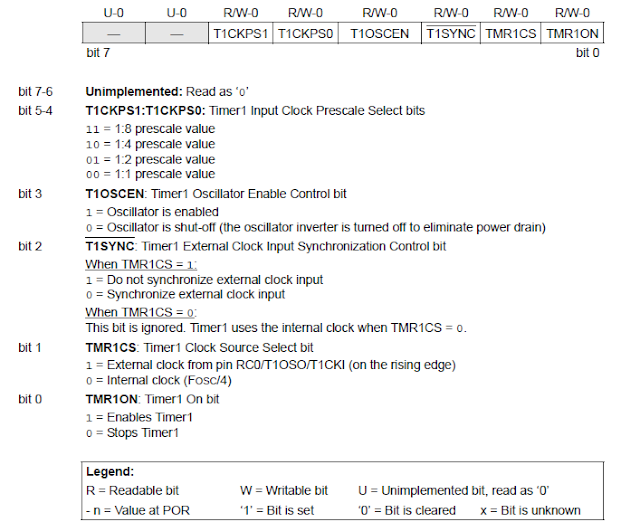## SERVO MOTOR CONTROL USING PIC16F877ASERVO MOTOR
Servo motors are used to control many things as it offers very precise rotation of 1 degree. For this it uses the feedback from a potentiometer connected.

For controlling the angle it require a pulse of certain duty cycle which can be achieved using the pulse with modulation (PWM) signal.
1. For 0 degree it takes a 800us pulse (us = microseconds)
2. For 90 degree it takes a 1500us pulse
3. For 180 degree it takes a 2200us pulse
Note:- This pulse can vary with different model of servo motor.

### We can control is using the PIC16F877A

For this we have to understand the PWM working of the micro-controller.

PIC has overall 3 timers
• TIMER0
• TIMER1
• TIMER2

We will use the TIMER1 for generating the PWMRegister Associated with TIMER1 as a TIMER/COUNTERTIMER1 Control RegisterTIMER1 Block Diagram The prescaler value is set to 1:1 The Crystal frequency used is 20MHz You can connect the Bluetooth and send the command to control the angle of the servo motor. If the data received is 0 the motor rotates to 0 degree If the data received is 1 the motor rotates to 90 degree If the data received is 2 the motor rotates to 180 degree Note:- Compiler or the software used here is MikroC pro for PIC THE CODE: -
/*code for generating 800us, 1500us and 2200us pulse for controlling servo motor 0, 90 and 180 degree
 Send the code from the bluetooth to the PIC16F877A for conrolling the angles   Crystal oscillator connected is 20MHz   Author:- ASHISH KUMAR       */ char a;             //char for recieving data from bluetooth unsigned lval=0x60; unsigned hval=0xF0; void interrupt(){   if(TMR1IF_bit){      PORTB.F0=!PORTB.F0;      TMR1L=lval;      TMR1H=hval;      TMR1IF_bit=0;   }   if(PIR1.F5)  {     while(PIR1.F5==0);     a=RCREG;     if(a=='0'){       //for 800us pulse (0 degree)       lval=0x60;       hval=0xF0;     }     else if(a=='1'){  //for 1500us pulse (90 degree)       lval=0xB4;       hval=0xE2;     }     else if(a=='2'){      //for 2200us pulse (180 degree)       lval=0x08;       hval=0xD5;     }   } } void main(){   OPTION_REG= 0x87;   TRISB.F0=0x00;   T1CON.F5=0;   T1CON.F4=0;   TMR1L=lval;   TMR1H=hval;   TMR1IE_bit=1;   INTCON.GIE=1;   INTCON.PEIE=1;   TMR1ON_bit=1;   TRISC.F6=0;   TRISC.F7=1;   SPBRG=32;   TXSTA=0x20;   RCSTA=0x90;   PIE1.F5=1;   PIE1.F4=0;   while(1){     //write your code   } } SIMULATION OUTPUT: -Proteus SimulationsPulse Output800us Pulse1500us Pulse2200us Pulse